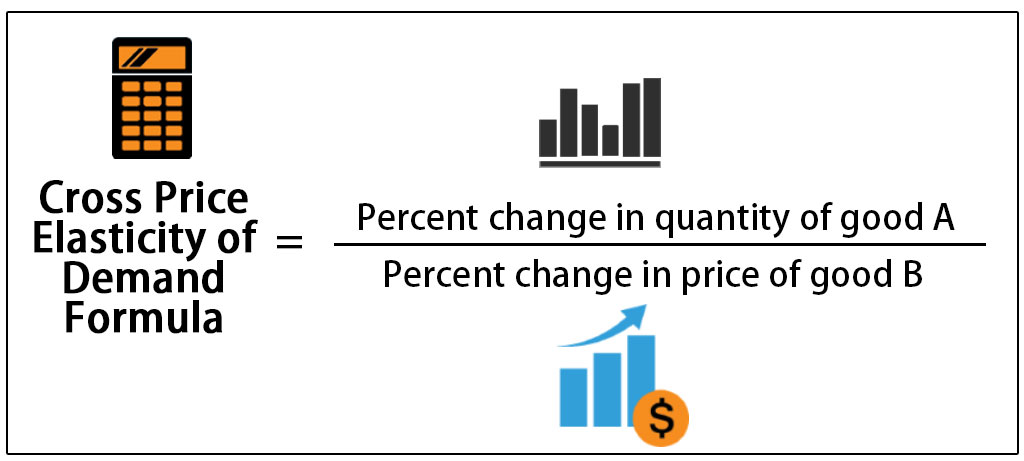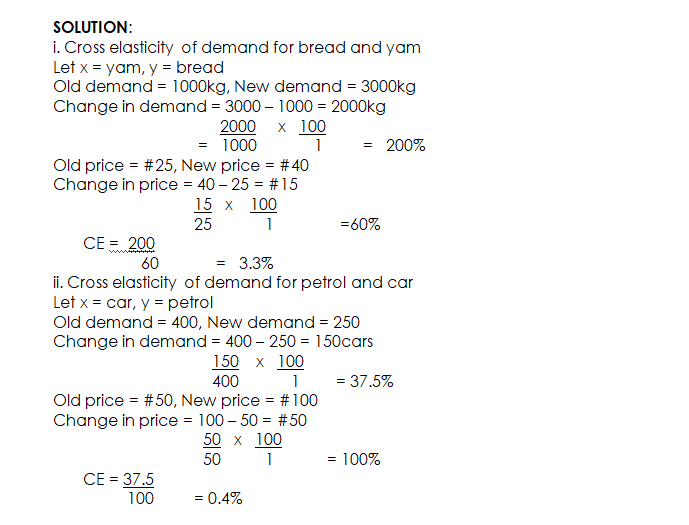# Cross Elasticity of Demand

Welcome to class!

In today’s class, we will be talking about the cross elasticity of demand. Enjoy the class!

### CROSS ELASTICITY OF DEMANDCONTENT

• Definition
• Types (Positive and Negative)
• Measurement of Income Elasticity of Demand

#### DEFINITION

Cross Elasticity of Demand is the degree of responsiveness of quantity demanded of commodity X to a little change in the price of commodity Y.  Cross elasticity of demand applies mainly to goods that are close substitute as well as complementary goods. For example, the demand for Milo will increase as a result of an increase in the price of Bournvita, all other things being equal.

Mathematically, cross elasticity of demand can be expressed as

% change in quantity demanded of commodity X

% change in the price of commodity Y

##### TYPES OF CROSS ELASTICITY OF DEMAND
1. Positive Cross Elasticity of Demand: With substitute goods, the cross elasticity of demand is always positive, (i.e. greater than zero), which means it is Elastic. This positive relationship is high with close substitutes and low with substitutes not very close.
2. Negative Cross Elasticity of Demand: With complementary (or jointly demanded goods), e.g. car and petrol, the cross elasticity of demand is always negative (i.e. less than zero), which means it is Inelastic. Here, too, high negative cross elasticity of demand indicates that the goods involved are highly complementary and, vice versa, i.e., low negative cross elasticity of demand means that the goods concerned are not highly complementary.

EVALUATION

1. Briefly explain cross elasticity of demand
2. Differentiate between complementary goods and substitute goods in relation to cross elasticity of demand.

Illustration:

The table below shows the response of quantity demanded to changes in price for two pairs of commodities. Use the table to answer the questions that follow:

Commodities            Changes in            Commodities        Changes in Quantity

Price                                                           Demanded

Old#         New#                                          Old kg       New kg

Bread                         25             40                 Yam                      1000          3000

Litre of petrol             50           100                 Car                        400             250

Calculate the cross elasticity of demand for (i) Bread and Yam, (ii) Petrol and Car.

SOLUTION:

1. Cross elasticity of demand for bread and yam

Let x = yam, y = bread

Old demand = 1000kg, New demand = 3000kg

Change in demand = 3000 – 1000 = 2000kg

2000     x   100

=   1000             1         =   200%

Old price = #25, New price = #40

Change in price = 40 – 25 = #15

15   x    100

25            1               =60%

CE =  200

60             =   3.3%

1. Cross elasticity of demand for petrol and car

Let x = car, y = petrol

Old demand = 400, New demand = 250

Change in demand = 400 – 250 = 150cars

150    x   100

400            1        = 37.5%

Old price = #50, New price = #100

Change in price = 100 – 50 = #50

50   x   100

50          1            = 100%

CE = 37.5

100         = 0.4%EVALUATION

1. How would you deduce complementary goods from a calculation of cross elasticity?
2. WAEC June 2000 Question No 2.

In our next class, we will be talking about Price Control/Legislation.  We hope you enjoyed the class.

Should you have any further question, feel free to ask in the comment section below and trust us to respond as soon as possible.

Pass WAEC, JAMB, NECO, BECE In One Sitting HERE!